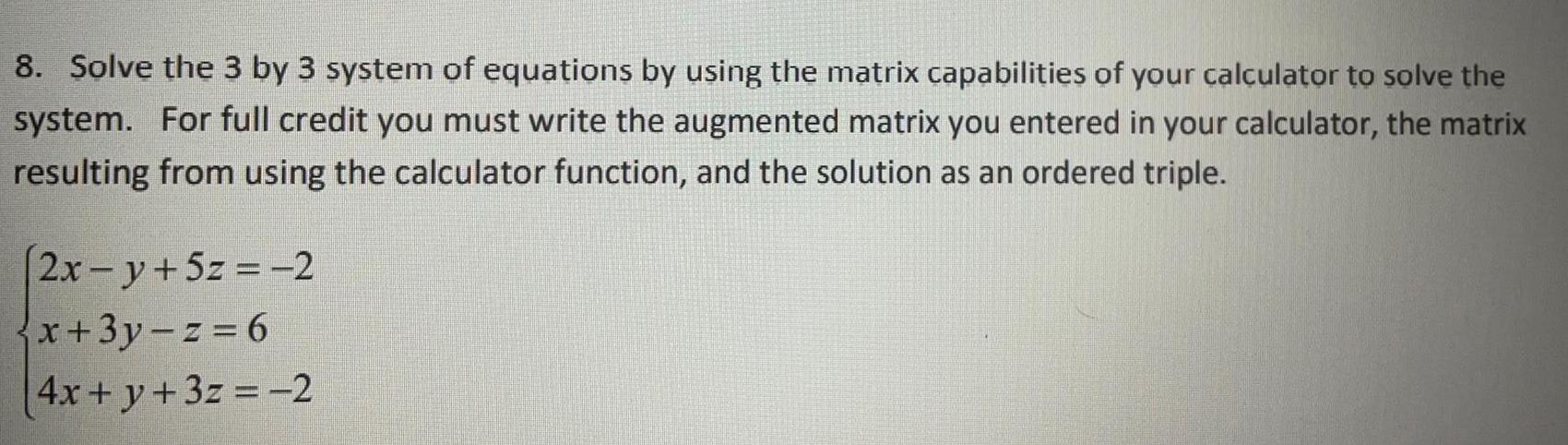Question:

# Solve the 3 by 3 system of equations by using the matrix

Last updated: 7/28/2022Solve the 3 by 3 system of equations by using the matrix capabilities of your calculator to solve the system. For full credit you must write the augmented matrix you entered in your calculator, the matrix resulting from using the calculator function, and the solution as an ordered triple. 2x -y + 5z = -2 x+3y-z=6 4x + y + 3z = -2